# Reduced Order Modeling of a Nonlinear Dynamical System as an Identified Linear Parameter Varying Model

This example shows reduced order modeling (ROM) of a nonlinear system using a linear parameter varying (LPV) modeling technique. The nonlinear system used to describe the approach is a cascade of nonlinear mass-spring-damper systems. The development of the reduced order model is based on the idea of obtaining an array of linear models over the operating range of the nonlinear model by means of linearization or linear model identification. These local linear models are stitched together using interpolation techniques to produce a linear parameter-varying representation. Such representations are useful for generating faster-simulating proxies as well as for control design.

For the purpose of local model computation, the operating space is characterized by a set of scheduling parameters (p1, p2 in the diagram below). These parameters are varied over a grid of pre-chosen values. An equilibrium operating condition corresponding to each grid point is first determined by a model trimming exercise (see `findop` in Simulink Control Design™). At each operating point, local perturbation experiments are performed to obtain a linear approximation of the system dynamics. The local models thus obtained are used to create a grid-based LPV model. This example requires that all the local models have matching structures. In particular, they must all use same state definitions. This example uses a Proper Orthogonal Decomposition (POD) approach to project the measured local responses into a common lower-dimensional space. The projected state trajectories are used to create lower-order state-space models using Dynamic Mode Decomposition (DMD).The example uses a nonlinear mass-spring-damper Simulink model as the reference high-fidelity system. This system exhibits nonlinear stiffness and damping forces; such forces are polynomial functions of the mass displacement. The development of the LPV model follows these steps:

1. The nonlinear model is trimmed over a range of displacement values.

2. At each trim point, local small-signal simulations are performed and the data recorded.

3. These datasets are then used to create an array of linear state-space models. One-degree-of-freedom linear parameter varying model is simulated along a specific trajectory of the force $\mathit{F}$. Multi-degree-of-freedom reduced order model is then obtained. The `n4sid` command, which estimates a linear state space model from input-output data, is used to identify linear models for the equilibrium points of the cascade of mass-spring-damper systems.

### Mass-Spring-Damper Model

Consider the single mass-spring-damper system (MSD) shown in the figure below, where $\mathit{m}$ is the mass, $\mathit{b}$ is the damping coefficient, $\mathit{k}$ is the stiffness coefficient of the spring, $\mathit{p}$ is the displacement of the mass from the equilibrium position, and $\mathit{F}$ and $\mathit{u}$ are input forces acting on the mass. $\mathit{F}$ represents a measurable disturbance and $\mathit{u}$ is a controllable input.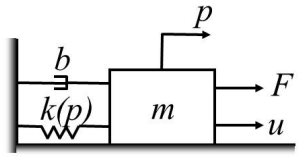The MSD system can be modeled as in :

`$m\underset{}{\overset{¨}{p}}=F+u-k\left(p\right)-b\left(\underset{}{\overset{˙}{p}}\right)$`

where $k\left(p\right)$ is the force due to spring stiffness and $b\left(\underset{}{\overset{˙}{p}}\right)$ the damping force. Using the displacement $p$ and velocity $v=\underset{}{\overset{˙}{p}}$ as state variables, this equation can be put in the state-space form:

`$\frac{d}{dt}\left[\begin{array}{c}p\\ v\end{array}\right]=\left[\begin{array}{c}v\\ \frac{1}{m}\left(F+u-b\left(v\right)-k\left(p\right)\right)\end{array}\right]$`

Defining $\mathit{x}\left(\mathit{t}\right)={\left[\mathit{p}\left(\mathit{t}\right)\text{\hspace{0.17em}\hspace{0.17em}\hspace{0.17em}}\mathit{v}\left(\mathit{t}\right)\right]}^{\mathit{T}}$ and discretizing the above equation yields

$x\left({t}_{k+1}\right)=x\left({t}_{k}\right)+\Delta t\left[\begin{array}{c}v\left({t}_{k}\right)\\ \frac{F\left({t}_{k}\right)+u\left({t}_{k}\right)-b\left(v\left({t}_{k}\right)\right)-k\left(p\left({t}_{k}\right)\right)}{m}\end{array}\right]$.

Simplify the notation by using ${x}_{k}$ for $x\left({t}_{k}\right)$ leading to:

`${x}_{k+1}={x}_{k}+\Delta t\left[\begin{array}{c}{v}_{k}\\ \frac{{F}_{k}+{u}_{k}-b\left({v}_{k}\right)-k\left({p}_{k}\right)}{m}\end{array}\right]$`

In this example, we consider the nonlinearity in the stiffness and the damping forces described by polynomials:

$k\left(p\right)={k}_{1}p+{k}_{2}{p}^{3}$,

and the damping coefficient given by

$b\left(v\right)={b}_{1}v+{b}_{2}{v}^{3}$,

where ${\mathit{k}}_{2}>0$ (stiffening spring). Let $\rho \text{\hspace{0.17em}}\left({\mathit{t}}_{\mathit{k}}\right)={\rho }_{\mathit{k}}$ denote a time-varying operating condition that you use for scheduling the nonlinear dynamics. For this example, you schedule the behavior on different values of the disturbance force, that is, ${\rho }_{\mathit{k}}={\mathit{F}}_{\mathit{k}}$. If $u\equiv 0$ and the disturbance force $F$is held constant at $F\equiv \rho$ then the mass moves to an equilibrium position $\underset{}{\overset{‾}{p}}$. This equilibrium position can be determined by solving the cubic equation $\rho =k\left(\underset{}{\overset{‾}{p}}\right)$ for $\underset{}{\overset{‾}{p}}$. Note that the damping force is zero at the equilibrium. Thus a parameterized collection of equilibrium conditions for the mass spring damper system is:

`$\left(\underset{}{\overset{‾}{x}}\left(\rho \right),\underset{}{\overset{‾}{u}}\left(\rho \right)\right)\doteq \left(\left[\begin{array}{c}\underset{}{\overset{‾}{p}}\\ 0\end{array}\right],0\right)$`

Linearizing this equation about the equilibrium conditions corresponding to a fixed value of $\rho$ yields:

${x}_{k+1}\approx \underset{}{\overset{‾}{{x}_{k+1}}}+A\left({\rho }_{k}\left({x}_{k}-\underset{}{\overset{‾}{{x}_{k}}}\right)+B\left({\rho }_{k}\right)\left({u}_{k}-\underset{}{\overset{‾}{{u}_{k}}}\right)$.

where:

`$\underset{}{\overset{‾}{{x}_{k}}}=\underset{}{\overset{‾}{x}}\left({\rho }_{k}\right),\underset{}{\overset{‾}{{u}_{k}}}=\underset{}{\overset{‾}{u}}\left({\rho }_{k}\right),A\left(\rho \right)=I+\Delta t\left[\begin{array}{ccc}0& & 1\\ -{k}_{lin}\left(\rho \right)/m& & -{b}_{lin}/m\end{array}\right],B\left(\rho \right)=\Delta t\left[\begin{array}{c}0\\ 1/m\end{array}\right]$`

The linearized spring constant at the operating condition $\rho$ is ${k}_{lin}\left(\rho \right)={k}_{1}+3{k}_{2}\underset{}{\overset{‾}{p}}\left(\rho {\right)}^{2}$. Similarly, ${b}_{lin}\left(\rho \right)={b}_{1}$ (since zero velocity at equilibrium). For more details, see section 4.2 in .

The model considered for simulation in this example is a cascade of 100 masses connected with springs and dampers as shown in the figure above.

### Local Analysis Using Perturbation Experiments

Set the values of the parameters required to simulate the MSD system. We consider $\mathit{m}=1\text{\hspace{0.17em}}\mathrm{kg}$, ${\mathit{k}}_{1}=0.5\text{\hspace{0.17em}}\mathit{N}/\mathit{m}$, ${\mathit{k}}_{2}=1$${\mathit{N}/\mathit{m}}^{3}$, ${\mathit{b}}_{1}=1\text{\hspace{0.17em}}N/\left(m/s\right),\text{\hspace{0.17em}}{\mathit{b}}_{2}=0.2\text{\hspace{0.17em}}\mathit{N}/{\left(\mathit{m}/\mathit{s}\right)}^{3}$.

```% System parameters M = 100; % Number of masses m = 1; % Mass of individual blocks, kg k1 = 0.5; % Linear spring constant, N/m k2 = 1; % Cubic spring constant, N/m^3 b1 = 1; % Linear damping constant, N/(m/s) b2 = 0.1; % Cubic damping constant, N/(m/s)^3 % Model parameters Tf = 20; % simulation time dt = 0.01; % simulation time step (fixed step)```

#### Experimental Setup

A perturbation experiment is performed on the MSD system with 100 masses as described in . First, a collection of operating points are defined for the MSD system. Then, data is collected by running the simulation for each operating point.

```% Initial condition % (needed to update the size of x in mdl_NL) x0 = zeros(M*2,1); % Simulation times dt = 0.1; % Time step, sec Tf = 20; tin = 0:0.1:Tf; % Time vector for snapshots, sec % Select Parameter Grid F_vec = (0:0.2:2)'; dut = [0 0]; dFt = [0 0]; F = F_vec(1); U0 = 0; Ngrid = numel(F_vec); Nt = numel(tin); % Batch trimming params(1).Name = 'F'; params(1).Value = F_vec;```

Create operating point specifications for the Simulink `MSD_NL.slx` model.

```opspec = operspec('MSD_NL'); opopt = findopOptions('DisplayReport','off'); op = findop('MSD_NL',opspec, params, opopt);```

Collect data at each grid point.

```% Collect data at each grid point xtrim = zeros(2*M,Ngrid); dXall = zeros(2*M,Nt,Ngrid); dUall = zeros(1,Nt,Ngrid); dYall = zeros(1,Nt,Ngrid); xOff = zeros(2*M,1,Ngrid); yOff = zeros(1,1,Ngrid); for i=1:Ngrid % Grab current grid point F = F_vec(i); U0 = 0; xtrim(:,i) = op(i).States.x; % Excite nonlinear system with chirp input, u w0 = 0.1; wf = 1+F; win = w0*(wf/w0).^(tin/Tf); uin = 1e-1*sin( win.*tin ); dut = [tin(:) uin(:)]; dFt = [0, 0; Tf, 0]; % Simulate using Simulink model x0 = op(i).States.x; simout_NL_perturb = sim('MSD_NL'); states = simout_NL_perturb.simout.Data; states = (squeeze(states))'; % Collect snapshots dXall(:,:,i) = states'-repmat(x0,[1 Nt]); dUall(:,:,i) = uin; % note that the 100th state is treated as model output dYall(:,:,i) = dXall(M,:,i); % Collect offset data (input offset is 0) xOff(:,:,i) = op(i).States.x; yOff(:,:,i) = op(i).States.x(M); end```

### Reduced-Order Linear Parameter Varying Modeling

Perform reduced-order modeling using linear parameter varying models, where the local models are obtained using Dynamic Mode Decomposition (DMD).

```X = dXall; Y = dYall; U = dUall; Nx = size(X,1); Nu = size(U,1); Ny = size(Y,1); Ngrid = size(X,3);```

Concatenate the state trajectories from individual grid points along time, for PCA-type projection of the states.

```% Concatenate the Ngrid (=1) state trajectories % along the time (second) dimension. X0all = reshape(X(:,1:end-1,:),Nx,[]); % Plot a few for verification plot(X0all([100 200],:)')```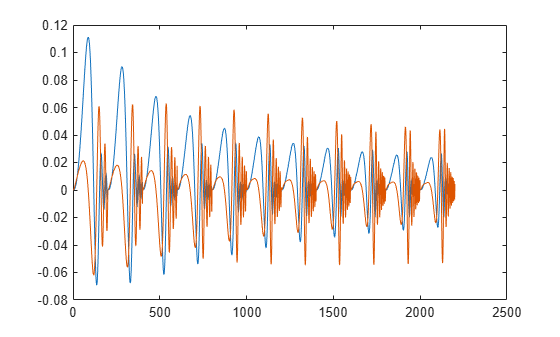```% POD modes using all state snapshots [UU,SS,~] = svd(X0all);```

Analyze the singular values in `SS` to determine a suitable projection dimension.

```bar(diag(SS)) xlim([0 10]) ylabel('Singular values') xlabel('Order')```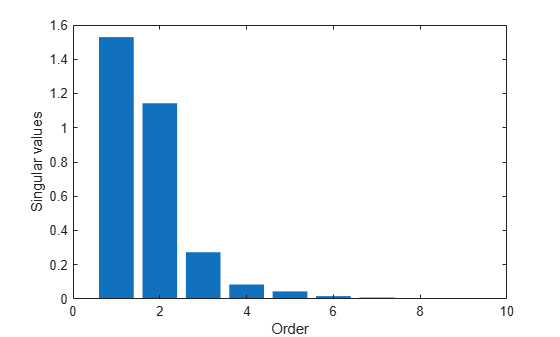The plot suggests, roughly, a fifth order model. Project the state trajectories obtained over the grid into a 5-dimensional space.

```% Select modes for subspace projection Q = UU(:,1:5); % Project all the state trajectories into the 5-dimensional space Xs = pagemtimes(Q',X);```

The projected state data `Xs` and the corresponding input and output trajectories at each grid point can be used in an identification algorithm to create the local linear models. In this example, we pose the identification problem as one of performing one-step prediction of the state and output trajectories:

`$\begin{array}{l}{\mathit{X}}_{\mathit{s}}\left(\mathit{t}+1\right)=\mathit{A}\text{\hspace{0.17em}}{\mathit{X}}_{\mathit{s}}\left(\mathit{t}\right)+\mathit{B}\text{\hspace{0.17em}}\mathit{U}\left(\mathit{t}\right)\\ \mathit{y}\left(\mathit{t}\right)=\mathit{C}\text{\hspace{0.17em}}{\mathit{X}}_{\mathit{s}}\left(\mathit{t}\right)\text{\hspace{0.17em}}+\mathit{D}\text{\hspace{0.17em}}\mathit{U}\left(\mathit{t}\right)\end{array}$`

Here, ${\mathit{X}}_{\mathit{s}}\left(\mathit{t}\right)$ and $\mathit{U}\left(\mathit{t}\right)$ are measured quantities. Hence the values of the unknowns $\mathit{A},\mathit{B},\mathit{C},\mathit{D}$ can be obtained by linear regression. This scheme is a form of dynamic mode decomposition (DMD) that is often employed for the study of fluid dynamics - the DMD modes and eigenvalues describe the dynamics observed in the time series in terms of oscillatory components. The DMD technique is used to obtain separate linear models at each grid point.

```% Generate values for Xs(t+1), and Xs(t) over a time span % Time is along the second dimension in the variables Xs, Y, U Xs_future = Xs(:,2:end,:); % Xs(t+1) Xs_current = Xs(:,1:end-1,:); % Xs(t) Y_current = Y(:,1:end-1,:); % Y(t) U_current = U(:,1:end-1,:); % U(t) H = cat(1,Xs_future,Y_current); % response to be predicted R = cat(1,Xs_current,U_current); % regressor data ("predictor") % Compute parameter estimates by linear regression ABCD = pagemrdivide(H,R); A = ABCD(1:5,1:5,:); B = ABCD(1:5,6:end,:); C = ABCD(6:end,1:5,:); D = ABCD(6:end,6:end,:); % Create a state space model array % to represent the dynamics over the entire grid Gred = ss(A,B,C,D,dt)```
```Gred(:,:,1,1) = A = x1 x2 x3 x4 x5 x1 0.9307 -0.1405 0.1308 -0.04535 0.1847 x2 0.005262 0.9328 0.1085 -0.04841 0.2236 x3 0.03029 0.03163 0.721 -0.05255 -0.2494 x4 -0.009416 -0.001662 0.1192 0.9586 0.119 x5 0.004583 -0.003053 -0.06434 0.06371 0.8054 B = u1 x1 0.06111 x2 0.08376 x3 -0.0544 x4 0.01752 x5 -0.01188 C = x1 x2 x3 x4 x5 y1 -0.7091 0.5734 -0.1015 -0.3294 -0.1513 D = u1 y1 -0.0006497 Gred(:,:,2,1) = A = x1 x2 x3 x4 x5 x1 0.9419 -0.1464 0.06915 -0.002446 -0.1135 x2 0.02028 0.925 0.02638 0.007876 -0.1765 x3 0.01989 0.03834 0.7779 -0.09571 0.03108 x4 -0.006492 -0.003484 0.1016 0.9706 0.04021 x5 0.002786 -0.001083 -0.05163 0.05412 0.8554 B = u1 x1 0.04756 x2 0.06568 x3 -0.04181 x4 0.01373 x5 -0.009241 C = x1 x2 x3 x4 x5 y1 -0.7083 0.5736 -0.0996 -0.3304 -0.1683 D = u1 y1 -0.0007994 Gred(:,:,3,1) = A = x1 x2 x3 x4 x5 x1 0.9499 -0.1462 0.05179 -0.01431 -0.1873 x2 0.0311 0.9257 0.004102 -0.01003 -0.2778 x3 0.01238 0.0393 0.796 -0.08962 0.09805 x4 -0.004367 -0.00373 0.09556 0.9663 0.01651 x5 0.001393 3.542e-05 -0.04495 0.05668 0.8758 B = u1 x1 0.04288 x2 0.05957 x3 -0.03709 x4 0.01222 x5 -0.007768 C = x1 x2 x3 x4 x5 y1 -0.7078 0.5742 -0.09622 -0.3255 -0.157 D = u1 y1 -0.0004653 Gred(:,:,4,1) = A = x1 x2 x3 x4 x5 x1 0.9562 -0.1463 0.03378 -0.04754 -0.1469 x2 0.0398 0.9258 -0.01917 -0.05789 -0.2245 x3 0.006522 0.0407 0.8161 -0.06638 0.05558 x4 -0.002751 -0.004067 0.09005 0.9572 0.02242 x5 0.0004756 0.001185 -0.03898 0.05992 0.877 B = u1 x1 0.04051 x2 0.05659 x3 -0.03429 x4 0.01141 x5 -0.006753 C = x1 x2 x3 x4 x5 y1 -0.7072 0.5746 -0.09611 -0.3229 -0.1364 D = u1 y1 -0.0003702 Gred(:,:,5,1) = A = x1 x2 x3 x4 x5 x1 0.9614 -0.1484 0.01434 -0.06714 -0.1018 x2 0.04691 0.9234 -0.04491 -0.0886 -0.1615 x3 0.001941 0.04416 0.8365 -0.06032 0.01742 x4 -0.001476 -0.004904 0.08478 0.953 0.02818 x5 -0.0001291 0.002709 -0.03445 0.05635 0.8835 B = u1 x1 0.03808 x2 0.05348 x3 -0.03152 x4 0.01065 x5 -0.005888 C = x1 x2 x3 x4 x5 y1 -0.7066 0.5747 -0.09782 -0.3217 -0.116 D = u1 y1 -0.0004601 Gred(:,:,6,1) = A = x1 x2 x3 x4 x5 x1 0.9657 -0.1518 -0.001988 -0.07121 -0.07759 x2 0.05295 0.9193 -0.06695 -0.09827 -0.125 x3 -0.001813 0.04873 0.8525 -0.06943 0.005651 x4 -0.0004053 -0.005972 0.08083 0.9529 0.02848 x5 -0.0005904 0.004249 -0.03202 0.04991 0.896 B = u1 x1 0.03564 x2 0.05025 x3 -0.02903 x4 0.009959 x5 -0.0053 C = x1 x2 x3 x4 x5 y1 -0.7062 0.5745 -0.1001 -0.3194 -0.1006 D = u1 y1 -0.0006534 Gred(:,:,7,1) = A = x1 x2 x3 x4 x5 x1 0.9695 -0.1555 -0.01425 -0.06818 -0.06976 x2 0.05831 0.9145 -0.08387 -0.09761 -0.1105 x3 -0.005053 0.05357 0.8635 -0.08334 0.01073 x4 0.0005459 -0.007078 0.07826 0.9541 0.02554 x5 -0.0009801 0.005636 -0.03138 0.04406 0.9096 B = u1 x1 0.03354 x2 0.04739 x3 -0.02712 x4 0.009419 x5 -0.004996 C = x1 x2 x3 x4 x5 y1 -0.7059 0.574 -0.1024 -0.3161 -0.09016 D = u1 y1 -0.0008752 Gred(:,:,8,1) = A = x1 x2 x3 x4 x5 x1 0.973 -0.1595 -0.02314 -0.06372 -0.07356 x2 0.0632 0.9095 -0.09646 -0.09424 -0.1122 x3 -0.007947 0.05839 0.8705 -0.09631 0.02561 x4 0.00142 -0.008168 0.07676 0.9553 0.02061 x5 -0.00133 0.006876 -0.03199 0.03982 0.9225 B = u1 x1 0.03217 x2 0.04546 x3 -0.0261 x4 0.009117 x5 -0.004979 C = x1 x2 x3 x4 x5 y1 -0.7057 0.5735 -0.1045 -0.3123 -0.08321 D = u1 y1 -0.001079 Gred(:,:,9,1) = A = x1 x2 x3 x4 x5 x1 0.9763 -0.1633 -0.02965 -0.06003 -0.08363 x2 0.06775 0.9045 -0.1059 -0.09133 -0.123 x3 -0.01059 0.06302 0.8748 -0.1067 0.04469 x4 0.00224 -0.00921 0.07596 0.9559 0.01489 x5 -0.00166 0.007997 -0.03333 0.03708 0.934 B = u1 x1 0.03147 x2 0.0444 x3 -0.02582 x4 0.009011 x5 -0.005168 C = x1 x2 x3 x4 x5 y1 -0.7056 0.5729 -0.1065 -0.3086 -0.07852 D = u1 y1 -0.001238 Gred(:,:,10,1) = A = x1 x2 x3 x4 x5 x1 0.9793 -0.167 -0.03448 -0.05721 -0.09453 x2 0.07204 0.8997 -0.1132 -0.08919 -0.1356 x3 -0.01305 0.06743 0.8773 -0.1148 0.06285 x4 0.00302 -0.01019 0.07563 0.9562 0.009657 x5 -0.00198 0.009019 -0.03512 0.03535 0.9436 B = u1 x1 0.03092 x2 0.04355 x3 -0.02575 x4 0.008942 x5 -0.0054 C = x1 x2 x3 x4 x5 y1 -0.7054 0.5723 -0.1082 -0.305 -0.07519 D = u1 y1 -0.001362 Gred(:,:,11,1) = A = x1 x2 x3 x4 x5 x1 0.9822 -0.1706 -0.03806 -0.05535 -0.1048 x2 0.07611 0.8951 -0.1188 -0.08809 -0.1477 x3 -0.01538 0.07164 0.8784 -0.1212 0.07885 x4 0.003773 -0.01113 0.07563 0.9561 0.005102 x5 -0.002301 0.009974 -0.03716 0.03431 0.9515 B = u1 x1 0.03044 x2 0.0428 x3 -0.02575 x4 0.008887 x5 -0.005636 C = x1 x2 x3 x4 x5 y1 -0.7054 0.5718 -0.1097 -0.3016 -0.0726 D = u1 y1 -0.001473 Sample time: 0.1 seconds 11x1 array of discrete-time state-space models. ```
```% Collect offset information % (obtained earlier during operating point search) Gred.SamplingGrid.F = F_vec; % scheduling parameter grid Gred_offset_x = pagemtimes(Q',xOff); Gred_offset_y = yOff; bodemag(Gred) % frequency responses of the 11 models```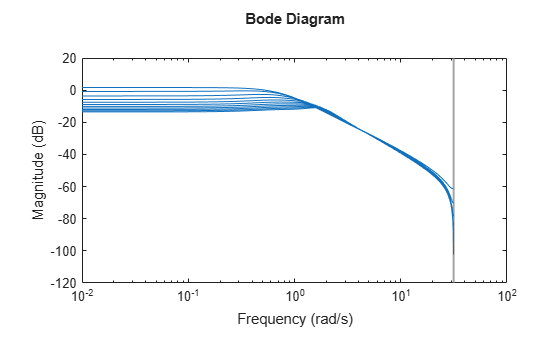The LTI array `Gred`, along with the offset data can be used to create an LPV model. There are two ways of doing so:

• In MATLAB using the `ssInterpolant` function to create an `lvpss` model

• In Simulink using the LPV System block

### LPV Model Creation and Simulation

Simulate the nonlinear and the linear parameter varying models with a sinusoidal input force and compare the results. First, simulate the original nonlinear system to generate the reference data.

```Tf = 50; tin = 0:dt:Tf; Nt = numel(tin); % Specify sinusoidal parameter (F) trajectory amp = -1; bias = 1; freq = 0.5; Ft = amp*cos(freq*tin)+bias; dFt = [tin(:) Ft(:)]; F = 0; % Specify input Force dut = [tin(:) zeros(Nt,1)]; dut(tin>=25,2) = 0.1; U0 = 0; % Simulate the high-fidelity nonlinear model simout_NL_MDOF = sim('MSD_NL'); tout = simout_NL_MDOF.tout; simout_NL_MDOF_data = squeeze(simout_NL_MDOF.simout.Data)';```

#### Simulate LPV Model in Simulink

Use the LPV System block to represent the LPV system. The block uses linear interpolation by default to interpolate the linear model matrices and the offsets.

```% Simulate ROM LPV open_system('MSD_MDOF_LPV')``````simout_NL_MDOF_red = sim('MSD_MDOF_LPV'); simout_NL_MDOF_red_data = squeeze(simout_NL_MDOF_red.simout.Data)'; ybar = simout_NL_MDOF_red_data(:,7);```

Compare the responses of the original (`MSD_NL`) and the LPV approximation (`MSD_MDOF_LPV`)

```% Compare the system output (position of the last mass) plot(tout, simout_NL_MDOF_data(:,M),'b',... tout, simout_NL_MDOF_red_data(:,6),'r-.',... tout, ybar,'g:') ylabel('Block 100 Position [m]'); xlabel('Time [sec]'); legend('Nonlinear model',... 'LPV (Simulink)',... 'Output offset (Trim)'); grid on;```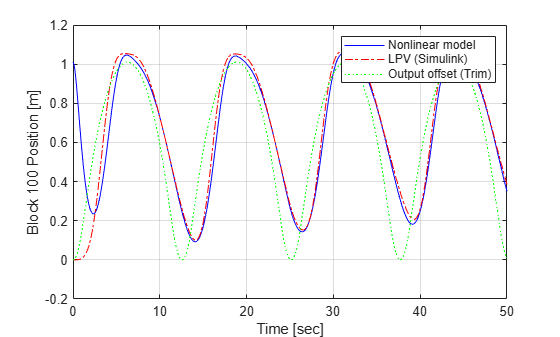#### Simulate LPV Model in MATLAB

The LPV model is encapsulated by the `lpvss` object in MATLAB. The `lpvss` object supports simulations, and model operations such as `c2d`, `feedback` connection etc. When the LPV model is composed of an array of local linear models, the `ssInterpolant` command can be used to create the LPV model.

```xc = squeeze(mat2cell(pagemtimes(Q',xOff),5,1,ones(1,Ngrid))); yc = squeeze(mat2cell(yOff,1,1,ones(1,Ngrid))); Offset = struct('dx',xc,'x',xc,'y',yc,'u',[]); LPVModel = ssInterpolant(Gred, Offset)```
```Discrete-time state-space LPV model with 1 outputs, 1 inputs, 5 states, and 1 parameters. ```

Simulate `LPVModel` using the same input and scheduling trajectories as used for original nonlinear system. The simulation is performed using the `lsim` command.

```Input = dut(:,2)+U0; Time = dut(:,1); Scheduling = dFt(:,2)+F; x0 = zeros(5,1); yLPV = lsim(LPVModel, Input, Time, x0, Scheduling); plot(tout, simout_NL_MDOF_data(:,M),'b',... Time, yLPV,'r-.') ylabel('Block 100 Position [m]'); xlabel('Time [sec]'); legend('Nonlinear model',... 'LPV (MATLAB)'); grid on;```The results show a good match between the original nonlinear system response, and that of its LPV approximation obtained by local linear modeling over a grid of scheduling values.

### References

 Jennifer Annoni and Peter Seiler. "A method to construct reduced‐order parameter‐varying models." International Journal of Robust and Nonlinear Control 27.4 (2017): 582-597.